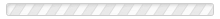Search:
(clear)
Results: 153
• ### i

• An imaginary number is one that includes the square root of -1, written √(-1). While one might think that such a number(...) Read More
• “Indeterminacy” or “quantum indeterminacy” refers to the uncertainty of results when measuring certain pairs of properties(...) Read More
• The “Indeterminacy Principle” is another name for the Heisenberg Uncertainty Principle (HUP). See the Heisenberg Uncertainty(...) Read More
• An interpretation of quantum mechanics describes the nature of reality in the quantum realm. Quantum mechanics is a set of(...) Read More
• An ion is formed when an atom gains or loses an electron. An atom which has lost one or more electrons has a positive(...) Read More
• To ionize is to convert a substance from atomic or molecular form to ions. An ion is a type of atom that has an imbalance(...) Read More
• Isotopes are a special kind of atom. Atoms with the same number of protons but different numbers of neutrons are isotopes of(...) Read More
• ### j

• A Joule is a unit for measuring energy. It's the amount of energy needed to lift a medium tomato one meter off the surface(...) Read More
• ### l

• The Law of Conservation of Energy says that the amount of energy in an isolated system remains constant. Energy cannot be(...) Read More
• The Law of Conservation of Matter was abandoned as inaccurate by physicists early in the 1900’s. For more information, see(...) Read More
• Linear momentum is the oomph something has due to having mass that’s in motion. If a football player were to ram into a(...) Read More
• “Lines of force” can best be explained with an illustration. The photo shows the lines of magnetic force created with a(...) Read More
• In everyday life, we assume the principle of locality. Here’s the principle of locality: Causing an effect on an object or(...) Read More
• Logical reasoning is reasoning that draws conclusions based on correct understanding of relationships. Here are examples of(...) Read More
• ### m

• The magnetic moment is the strength of the response of a piece of metal to a magnetic field. It is a measure of the degree(...) Read More
• The magnetic moment of an electron is symbolized as g and is called the “g-factor.” The magnetic moment is the degree to(...) Read More
• Pi and e are examples of mathematical constants. A mathematical constant has the same value no matter which mathematical(...) Read More
• (Also called “observation”). Measurement is of considerable consequence in the quantum world. At least in some(...) Read More
• Understanding the Measurement Problem requires understanding the unique role of measurement in quantum mechanics. The entry(...) Read More
• A metal is a kind of matter, usually derived from rocks found in nature. Examples of metals include iron, aluminum, and(...) Read More
• A minimal theory in physics is a theory that accounts for known phenomena with the simplest approach conceivable. Of course,(...) Read More
• “Moment,” as used in physics, means the amount of a force that rotates the object. The term “moment” is used in regard to a(...) Read More
• Moment of force is also called torque. “Moment,” as used in physics, means the amount of force experienced by an object due(...) Read More
• In classical physics, moment of momentum is the momentum or oomph which an object has as it rotates about an axis. It is the(...) Read More
• (Abbreviated p. Momentum is the oomph something has due to having mass that’s in motion. Let’s say you just happen to be(...) Read More
• ### n

• The principle of nonlocality in quantum mechanics says that quantum particles can “know” the states of other quantum(...) Read More
• Scientists call the everyday matter of our world, such as tables and chairs, “normal matter.” Normal matter is made up of(...) Read More
• ### o

• See measurement in quantum mechanics and see observer. Read More
• In quantum mechanics, the term "observer" is used in a specialized manner. It has a meaning quite different from that of the(...) Read More